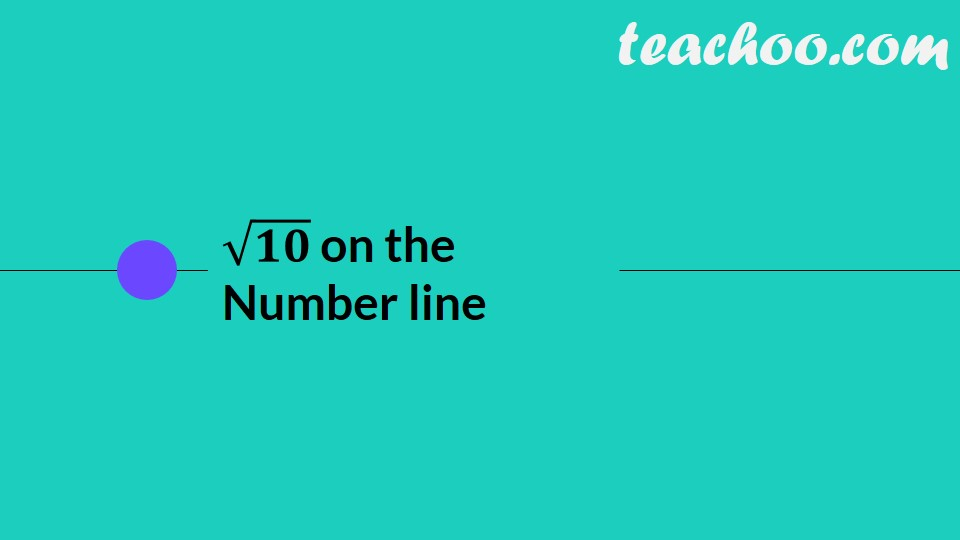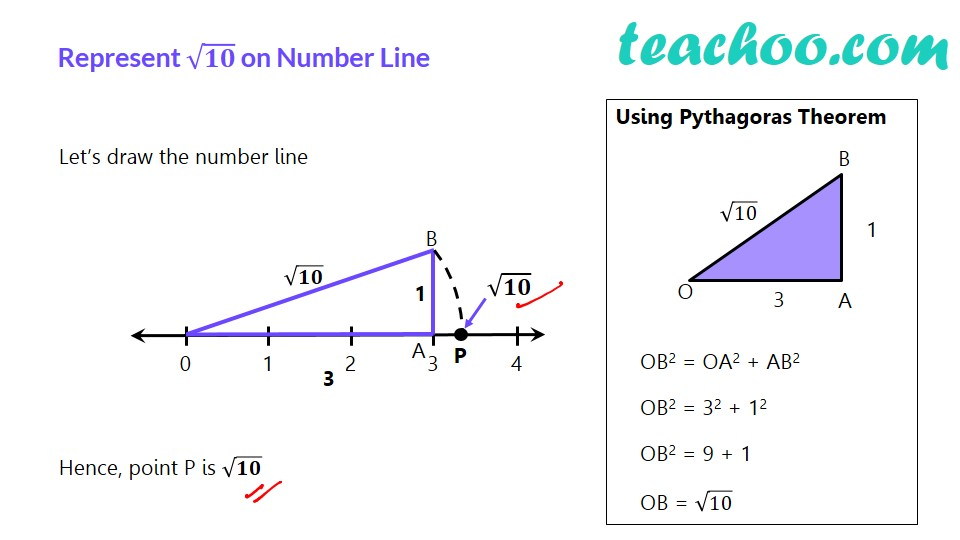Irrational Numbers

Chapter 1 Class 9 Number Systems
Concept wiseLearn in your speed, with individual attention - Teachoo Maths 1-on-1 Class

### Transcript

√𝟏𝟎 on the Number line Represent √𝟏𝟎 on Number Line Let’s draw the number line Hence, point P is √𝟏𝟎 Using Pythagoras Theorem OB2 = OA2 + AB2 OB2 = 32 + 12 OB2 = 9 + 1 OB = √10